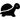## Overview

The MU-puzzle

A simple formal system (the MIU-system) is presented, and the reader is urged to work out a puzzle to gain familiarity with formal systems in general. A number of fundamental notions are introduced: string, theorem, axiom, rule of inference, derivation, formal system, decision procedure, working inside/outside the system. (p. viii)

### Questions

Many of these questions were adapted from Curry and Kelleher's lecture notes for this chapter.

1. In principle, any three letters could have been chosen for the puzzle. Why were “M”, “I”, and “U” employed?
2. What does it mean to be “in possession of a string”?
3. The text states that from MU you can get MUU. It's a true statement. Does that imply that you can get MU? If so, then the puzzle is solved!
4. How does a theorem differ from an axiom (both in mathematics and the sense used in GEB)?
5. You've probably become convinced that, when MI is the only axiom, all theorems will begin with M. How did you become convinced of that? Which mode of reasoning are you using -- M-mode, I-mode, or U-mode? Or if you're not convinced, why not?
6. Hofstadter suggests that the numbers 3 and 2 play important roles in the MIU-system. What roles?
7. Will the decision tree shown in Figure 11 (p. 46) produce every theorem of the MIU-system from the axiom MI?
8.Be sure to try the puzzle as stated! Is MU a theorem? Why or why not?
9.You've found a number of MIU-theorems that can be derived from MI. You can do the reverse with at least some of them: you can start from one of those theorems and derive MI. (Example: MIUU.) If a theorem x can be derived from MI and MI can be derived from x, let's call them "equivalent".
1. Are there theorems that aren't equivalent to MI?
2. Can you make rules that generate only theorems that are equivalent to MI?
10.Can you make a decision procedure for the MIU system, which takes in any string of M, I, and U and tells you definitively that it's a theorem or not a theorem? To do this, you have to do things besides just applying the rules, because the rules don't tell you about non-theorems.
1. Can you make another decision procedure that decides whether a string is equivalent to MI?

## Topics

### The MIU-system

(For example, here we might work through some examples of MIU-system derivations, and link to scripts in some programming languages that implement the system.)

### Working inside and outside of the system

(This is an important concept for understanding what the Tortoise's point is, in the following Two-Part Invention.)